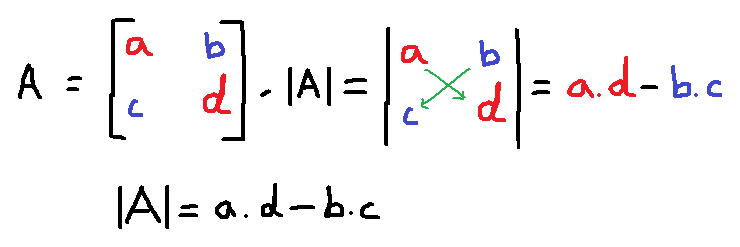# Finding Determinant By Cross MultiplicationIn the previous article, we have seen how Determinant decides whether a system of equation (read square matrix) has inverse, or it has a solution, only when the determinant is not zero. The determinant is obtained from the equation given below.$determinant = \sum \pm a_{1\alpha}a_{2\beta}...a_{nv}$

To know more about finding determinant in this way , read previous article. Here we will discuss about finding determinant by cross multiplication but before that let us understand the different notations used to represent determinants.

### Notation For Determinants

There are several notation for determinants given by earlier mathematicians. Suppose$A$ represents a augmented matrix from a system of linear equations, then determinant of$A$ is given below.

Let the matrix$A$ be a 2 x 2 matrix.$A = \begin{bmatrix}a_{11} & a_{12}\\a_{21} & a_{22}\end{bmatrix}$

Different ways to represent determinant of matrix$A$$det(A)$  -- (1)$|A|$ -- (2)$\begin{vmatrix}a_{11} & a_{12}\\a_{21} & a_{22}\end{vmatrix}$ -- (3)

### Determinant as a Function

Imagine determinant to a function that take a square matrix as input and give a single value as output. For example,$f(x) = x^3$ be a function where$x$ could be any real number. Similarly,$det(A)$ is a function that matrix as input and give a determinant value$d$. The determinant value is always integer because it is linear combination of integers, that is, all values are integers in the matrix.

Determinant of$1 \times 1$ Matrix

If$A$ is a matrix with just one element, then its determinant is the same element.

Example #1

Let$A$ be a square matrix of order$1 \times 1$$A = \begin{bmatrix}2\end{bmatrix}$

Then the determinant of$A$ is$|A| = |2| = 2$

### Determinant of$2 \times 2$ Matrix

The determinant of a$2 \times 2$ matrix is obtained by performing cross multiplication. See the following figure.Example #2

Let$A$ be a$2 \times 2$ square matrix. Find the determinant of the matrix$A$.

Solution:

Let the$A$ be 2 x 2 square matrix.$A = \begin{bmatrix}2 & 3\\1 & 5\end{bmatrix}$$|A| = a \times d - b \times c$$|A| = 2 \times 5 - 3 \times 1$$|A| = 10 - 3 = 7$

Example #3

Let$B$ be a square matrix of order$2 \times 2$. Find the determinant of the matrix$B$.

Solution:

Let$B$ be a square matrix of order$2 \times 2$.$B = \begin{bmatrix}5 & -1\\4 & -3\end{bmatrix}$$|B| = a \times d - b \times c$$|B| = 5 \times (-3) - (-1) \times 4$$|B| = (-15) - (-4)$$|B| = (-15) + 4 = -11$

### Determinant Of$3 \times 3$ Matrix

The determinant of a$3 \times 3$ matrix is also possible through cross multiplication; Since we have a larger matrix we need to convert the larger matrix into smaller matrix to compute determinant. See figure below.

The post Finding Determinant By Cross Multiplication appeared first on Notesformsc.

This post first appeared on Notesformsc, please read the originial post: here

# Share the post

Finding Determinant By Cross Multiplication

×